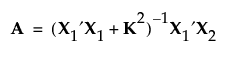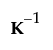Design of Experiments Guide > Technical Details > The Alias Matrix > Designs with If Possible Effects
Publication date: 11/10/2021

## Designs with If Possible Effects

For designs with If Possible effects (Bayesian D- and I-optimal designs), the alias matrix is given as follows:where K is a diagonal matrix with these values:

k = 0 for Necessary terms

k = 1 for If Possible main effects, powers, and interactions involving a categorical factor with more than two levels

k = 4 for all other If Possible terms

In the Bayesian case, the alias matrix gives the aliasing of effects corresponding to a ridge regression with a prior variance of. For additional detail on Bayesian designs, see Bayesian D-Optimality and Bayesian I-Optimality.

In the Custom Design platform, you can control the weights used for If Possible terms by selecting Advanced Options > Prior Parameter Variance from the red triangle menu. There you can set prior variances for all model terms by specifying the diagonal elements of K. The option updates to show the default weights when you click Make Design.